## Tuesday, 15 May 2012

### M2: Algebra and geometry Part 2

When the rate of change considering the segment length is considered, one of the direct application is mechanical deduction. Differentiation build up a strong relationship between displacement, velocity and acceleration so differential equation would be useful here.

Locus of a certain point in coordinate geometry is a big topic in core/M2 mathematics, and it has significant applications, remarkably some pivot systems.

Example 3a. (Locus on linear system) Let A, B and C be 3 points on positive x-axis, positive x-axis and negative x-axis respectively. AB = 5, BC = 7. Let |OA| = x, |OC| = y, find dy/dx.

What I want to illustrate here is that, the differentiation operator has a very nice behavior that allows us to do questions in many ways. Denote |OB| = h.

Solution 1. Set up relation between x,y through h.
Obviously there are pairs of right angled triangle, hence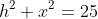and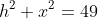, then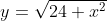(since y is positive), then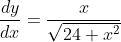.

Solution 2. Implicit differentiation.
First we set up the explicit relation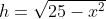and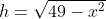. By differentiatingw.r.t. t,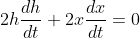, and do the same thing to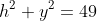to get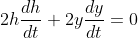.

Now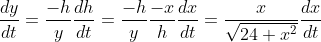Therefore. Note that we can multiply both sides by dt/dx, and eliminate the terms dt by chain rule.

Solution 3. Differentation through angle.
Let angle BAC be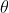. Then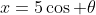,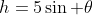,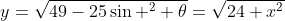. Now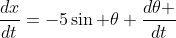,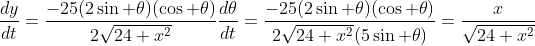.

The same question can be solved by different approaches, and that's the art of problem solving.

Example 3b. (Movement on circle) There are 3 points on the coordinate plane: A(7,k), B(6,0) and C(8,0) where k is a positive real number. E is the mid-pt of AB. Let C1 be the circle with center C and radius r1 = CE, intersecting AC at D (D distinct from E). Let C2 be the circle with center B and radius r2 = BD. C2 intersect the circle at origin with radius 5 at F where F has positive value. Disprove the claim that angle BOF holds a linear relationship against k by finding the relationship between angle BOF and k.

Sometimes the locus may not need to vary against the initial condition, but the speed of the point varies. We are not going to discuss the velocity of points and curvature stuffs here though.

With some sketching it looks like the claim is true but it can be easily rejected with a few trial on selected k. The only problem that we need to do is to find the relationship between the angleand k.

First we locate E at (6.5, k/2) by mid-point theorem. The equation of C1 can be written as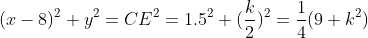. The line ac can be modelled byby point-slope form. Luckily we can directly put this into the circle equation: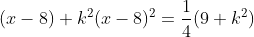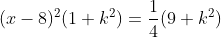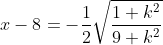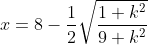Note that we take negative square root so that the x-value is between 7 and 8 and the y-value is positive, i.e., D is on AC not AC extended. Then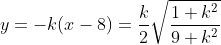.

Consider C2: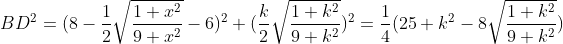, the equation of circle C2 is given by: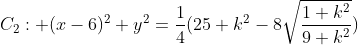. The circle at origin has equation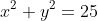, then the term y squared can be cancelled.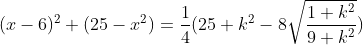, solving x gives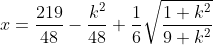.

The y-value of the intersection is not necessary to be calculated (and is extremely ugly). We have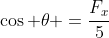and up to now we are enough to disprove the claim by the relationship set up. By sketching we have the graph:
Which is a bit concave but not far from linear stuffs in typical range of value.

Foods for thought.
1) Notice the behavior of the graph when k is small. By sketching when k is small C1 has no intersection with D hence the intersection point F should be undefined but the relationship itself is defined. Explain.
2) By (1) or otherwise, explain why the function stops at about x = 22. Find the two end-point of the graph by either geometrical or algebrical means, then redefine the relationship.
3) By extending the relation to negative k, show that the relationship is even.
4) The y-intercept of the graph is non-zero. Explain in terms of geometrical interpretation.

Example 4. (Angle variation) For triangle OAB, OA=OB, AB = 1. Y is a point on AB so that angle AOY : angle YOB = 1:n. Let AY = y.  Find the range of y.

Let the perpendicular foot from O to AB be OX, and |OX|=h. Let angle AOY be ﻿, then angle YOX is.

Consider triangle AOX we have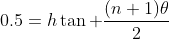, then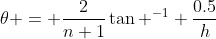.

Consider triangle XOY we have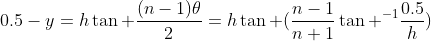.

Therefore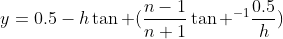. By considering the sign of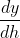we can show that the function is monotonic but we are not going to do this here. (It can also be shown easier in geometric visualization.) The function is also bounded because it is clear that y is between 0 and 0.5, therefore the limit to zero and infinity are the limits of y.

Firstly we take the limit to zero.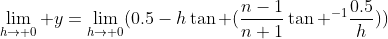. It is clear that the tangent term won't rage to infinity as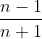is smaller than 1 while h tends to zero. Then the limit tends to 0.5. Now consider the limit to infinity. To make life easier we call the termas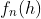, and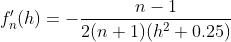. (differentiate w.r.t. h)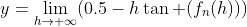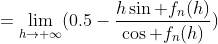, note that the denominator is 1 so we take it away: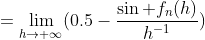, apply L' hopital rule: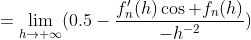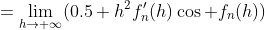, the cosine term appear again and is 1 so we take it away again: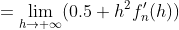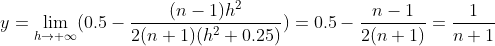Then 1/(n+1) < y < 0.5 can be concluded. This conclusion can be made by some less vigorous geometric proof: 1) When h tends to zero, Y shrinks to X so y tends to 0.5. 2) What h tends to infinity, it follows the small-angle approximation, tangent-angle-proportion ~ angle-proportion ~ segment proportion, hence the conclusion y tends to 1/(n+1). The above example demonstrated a possible way to sketch the behavior of figures when it tends to some singularities, and geometric questions can be transformed into algebra questions and are solved in a systemetical way. Note that the above approach applied many technique in handling indeterminated limits and they should be familirized by various students.

Foods for thought. II
Use non-calculus approach to show the case for 1:2 and 1:3. In fact, this is an examination question in HKDSE 2012 M2.

Algebra and Geometry Part I